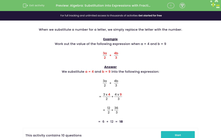# Practise Substitution into Expressions with Fractions

In this worksheet, students will substitute values into expressions with fractions.Key stage:  KS 3

Curriculum topic:   Algebra

Curriculum subtopic:   Substitute Numerical Values for Formulae/Expressions

Popular topics:   Algebra worksheets

Difficulty level:#### Worksheet Overview

When we substitute a number for a letter, we simply replace the letter with the number.

Example

Work out the value of the following expression when a = 4 and b = 9

 3a + 4b 2 3

We substitute a = 4 and b = 9 into the following expression:

 3a + 4b 2 3

 = 3 x 4 + 4 x 9 2 3

 = 12 + 36 2 3

=  6  +  12  =  18

Let's try some questions now.

### What is EdPlace?

We're your National Curriculum aligned online education content provider helping each child succeed in English, maths and science from year 1 to GCSE. With an EdPlace account you’ll be able to track and measure progress, helping each child achieve their best. We build confidence and attainment by personalising each child’s learning at a level that suits them.

Get started